Courses

# NCERT Solutions(Part - 2) - Ratio and Proportion Class 6 Notes | EduRev

## Class 6 : NCERT Solutions(Part - 2) - Ratio and Proportion Class 6 Notes | EduRev

The document NCERT Solutions(Part - 2) - Ratio and Proportion Class 6 Notes | EduRev is a part of the Class 6 Course Mathematics (Maths) Class 6.
All you need of Class 6 at this link: Class 6

Exercise 12.2
Question 1:
Determine if the following are in proportion.
(a) 15, 45, 40, 120
(b) 33, 121, 9, 96
(c) 24, 28, 36, 48
(d) 32, 48, 70, 210
(e) 4, 6, 8, 12
(f) 33, 44, 75, 100
(a)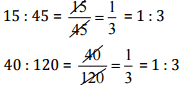Since 15:45 = 40:120
Therefore, 15,45,40,120 are in proportion.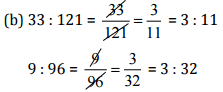Since 33:121 ≠ 9:96
Therefore. 33,121, 9,96 are not in proportion.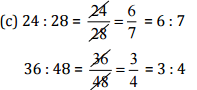Since 24 : 28 ≠ 36 : 48
Therefore, 24, 28,36, 48 are not in proportion.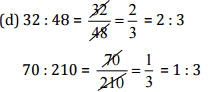Since 32:48 ≠ 70:210
Therefore, 32,48, 70, 210 are not in proportion.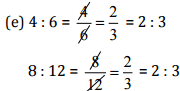Since 4:6 = 8:12
Therefore, 4, 6, 8,12 are in proportion.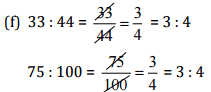Since 33:44 = 75:100
Therefore, 33, 44, 75,100 are in proportion.

Question 2:
Write True (T) or False (F) against each of the following statements:
(a) 16:24::20:30
(b) 21:6::35:10
(c) 12:18::28:12
(d) 8:9::24:27
(e) 5.2:3.9::3:4
(f) 0.9:0.36::10:4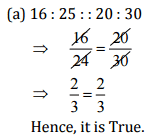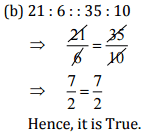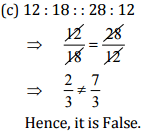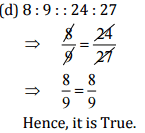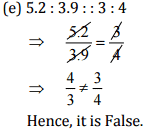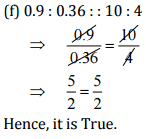Question 3:
Are the following statements true?
(a) 40 persons: 200 persons = Rs 15: Rs 75
(b) 7.5 litres: 15 litres = 5 kg: 10 kg
(c) 99 kg: 45 kg = Rs 44: Rs 20
(d) 32 m: 64 m = 6 sec: 12 sec
(e) 45 km: 60 km = 12 hours: 15 hours
(a) 40 persons: 200 persons =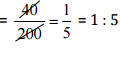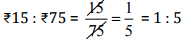Since, 40 persons : 200 persons = Rs 15 : Rs75
Hence, the statement is true.

(b) 7.5 litres : 15 litres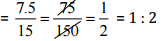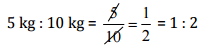Since, 7.5 litres : 15 litres = 5 kg : 10 kg
Hence, the statement is true.

(c)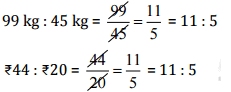Since, 99 kg: 45 kg = Rs 44 : Rs 20
Hence, the statement is true.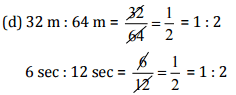Since, 32 m : 64 m = 6 sec : 12 sec
Hence, the statement is true.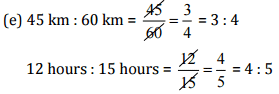Since, 45 km : 60 km ≠ 12 hours : 15 hours
Hence, the statement is not true.

Question 4:
Determine if the following ratios form a proportion. Also, write the middle terms and extreme terms where the ratios form a proportion.
(a) 25 cm: 1 m and Rs 40 : Rs 160
(b) 39 litres: 65 litres and 6 bottles: 10 bottles
(c) 2 kg: 80 kg and 25 g: 625 g
(d) 200 mL: 2.5 litre and Rs 4: Rs 50
(a) 25 cm : 1 m = 25 cm : (1 x 100) cm = 25 cm : 100 cm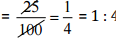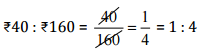Since the ratios are equal, therefore these are in proportion.
Middle terms = 1 m, Rs 40 and Extreme terms = 25 cm, Rs 160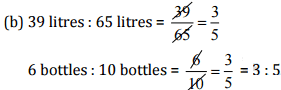Since the ratios are equal, therefore these are in proportion.
Middle terms = 65 litres, 6 bottles and Extreme terms = 39 litres, 10 bottles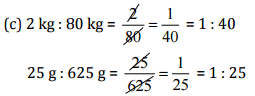Since the ratios are not equal, therefore these are not in proportion,

(d) 200 ml :2.5 litres = 200 ml:(25000) litres = 200 ml: 2500 ml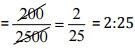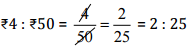Since the ratios are equal, therefore these are in proportion.
Middle terms = 2.5 litres, Rs 4 and Extreme terms = 200 ml, Rs 50

Exercise 12.3
Question 1:
If the cost of 7 m of cloth is Rs 294, find the cost of 5 m of cloth.
Cost of 7 m of cloth = Rs 294
∴ Cost of 1 m of cloth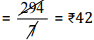Cost of 5 m of cloth = 42 x 5 = Rs 210
Thus, the cost of 5 m of cloth is Rs 210.

Question 2:
Ekta earns Rs 1500 in 10 days. How much will she earn in 30 days?
Earning of 10 days = Rs 1500
∴ Earning of 1 day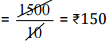∴ Earning of 30 days = 150 x 30 = Rs 4500
Thus, the earning of 30 days is Rs 4,500.

Question 3:
If it has rained 276 mm in the last 3 days, how many cm of rain will fall in one full week (7 days)? Assume that the rain continues to fall at the same rate.
Rain in 3 days = 276 mm
Rain in 1 day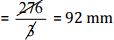∴ Rain in 7 days = 92x7 = 644 mm
As 1mm = 0.1cm
∴ 644mm = 64.4cm
Thus, the rain in 7 days is 64.4 cm.

Question 4:
Cost of 5 kg of wheat is Rs 30.50.
(a) What will be the cost of 8 kg of wheat?
(b) What quantity of wheat can be purchased in Rs 61?
(a) Cost of 5 kg of wheat = Rs 30.50
∴ Cost of 1 kg of wheat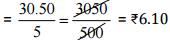∴ Cost of 8 kg of wheat = 6.10 x 8 = Rs 48.80

(b) From Rs 30.50, quantity of wheat can be purchased = 5 kg
∴ From Rs 1, quantity of wheat can be purchased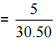From Rs 61, quantity of wheat can be purchase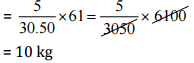Question 5:
The temperature dropped 15 degree Celsius in the last 30 days. If the rate of temperature drop remains the same, how many degrees will the temperature drop in the next ten days?
Degree of temperature dropped in last 30 days = 15 degrees
Temperature drop in 1 day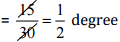Temperature drop in next 10 days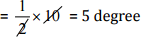So, there will be a temperature drop of 5° Celsius in next 10 days.

Question 6:
Shaina pays Rs 7500 as rent for 3 months. How much does she has to pay for a whole year, if the rent per month remains same?
Rent paid for 3 months = Rs 7500
∴ Rent paid for 1 month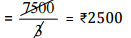∴ Rent paid for 12 months = 2500 x 12 = Rs 30,000
Thus, the total rent for one year is Rs 30,000.

Question 7:
Cost of 4 dozens bananas is Rs 60. How many bananas can be purchased for Rs 12.50?
Cost of 4 dozen bananas = Rs 60
Cost of 48 bananas = Rs 60    [4 dozen = 4 x 12 = 48]
∴From Rs 60, number of bananas which can be purchased = 48
From Rs 1, number of bananas which can be purchased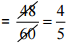From Rs 12.50, number of bananas which can be purchased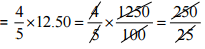=10 bananas
Thus, 10 bananas can be purchased for Rs 12.50.

Question 8:
The weight of 72 books is 9 kg what is the weight of 40 such books?
The weight of 72 books = 9 kg
The weight of 1 book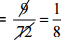∴ The weight of 40 books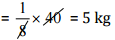Thus, the weight of 40 books is 5 kg.

Question 9:
A truck requires 108 litres of diesel for covering a distance of 594 km. How much diesel will be required by the truck to cover a distance of 1650 km?
For covering 594 km, a truck will be required diesel = 108 litres
∴ For covering 1 km, a truck will be required diesel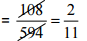For covering 1650 km, a truck will be required diesel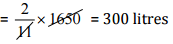Thus, 300 litres diesel is required by the truck to cover a distance of 1650 km.

Question 10:
Raju purchases 10 pens for Rs 150 and Manish buys 7 pens for Rs 84. Can you say who got the pen cheaper?
Raju purchase 10 pens for = Rs 150
∴ Raju purchases 1 pen for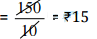Manish purchases 7 pens for = Rs 84
∴ Manish purchases 1 pen for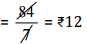Thus, Manish got the pens cheaper.

Question 11:
Anish made 42 runs in 6 overs and Anup made 63 runs in 7 overs. Who made more runs per over?
Anish made in 6 overs = 42 runs
Anish made in 1 overs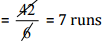∴ Anup made in 1 overs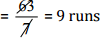Thus, Anup made more runs per over.

Offer running on EduRev: Apply code STAYHOME200 to get INR 200 off on our premium plan EduRev Infinity!

## Mathematics (Maths) Class 6

185 videos|229 docs|43 tests

,

,

,

,

,

,

,

,

,

,

,

,

,

,

,

,

,

,

,

,

,

;